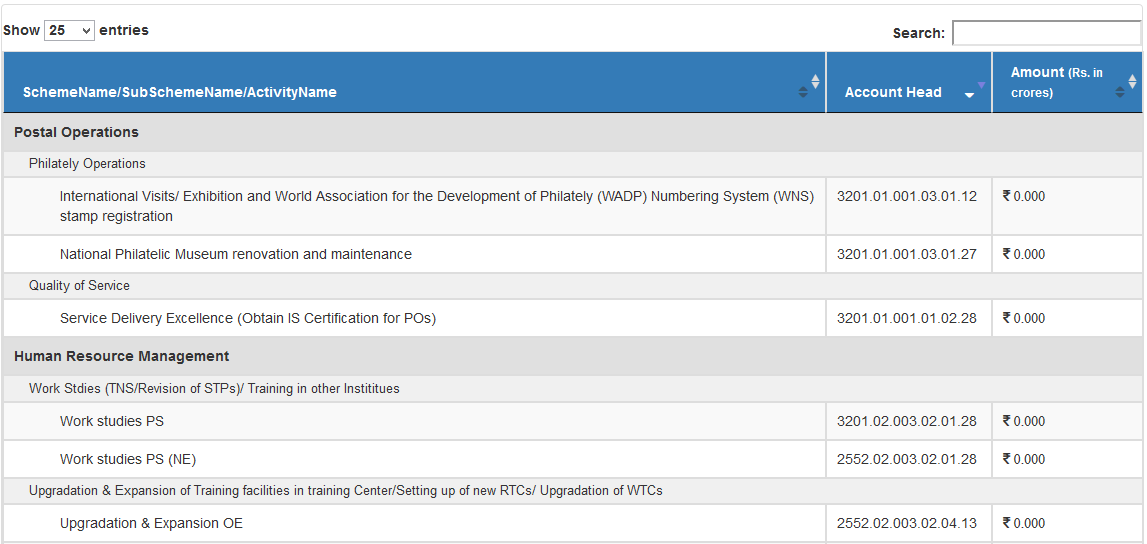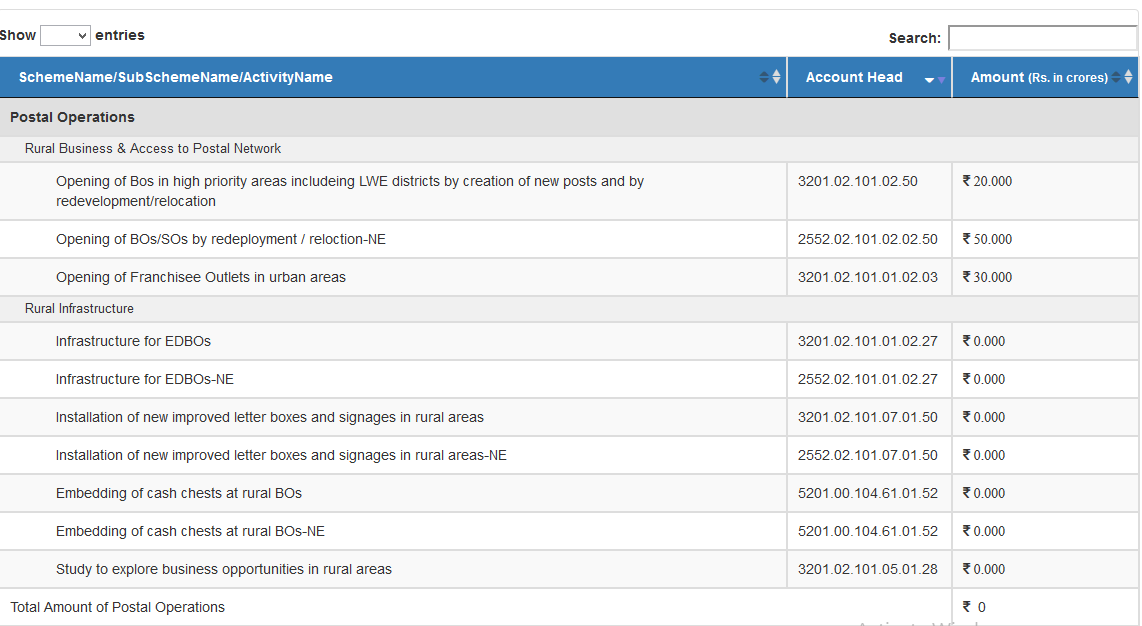# Dynamic Multi-Grouping

Hi dear,
I am new in datatable.I am stuck befor 5 days for creating this example dynamic.
https://datatables.net/extensions/rowgroup/examples/initialisation/multipleGroups.html

I have 3 table scheme,subscheme,activity in sql server and i want showing data like above example .
thanks.

Hi @Tyagi ,

We're happy to take a look, but as per the forum rules, please link to a test case - a test case that replicates the issue will ensure you'll get a quick and accurate response. Information on how to create a test case (if you aren't able to link to the page you are working on) is available here.

Cheers,

Colin

• Thanks

• edited November 2019

Hi @colin ,

My need like this

https://datatables.net/extensions/rowgroup/examples/initialisation/multipleGroups.html

first shows the Scheme name

after SubScheme Name
and after Amount.

Here is my code.

``````        <table id="example" class="table table-striped table-bordered" style="width:100%">
<tr>

@*<th>Sub-Schem </th>*@
<th>Activity</th>
<th>Schem </th>
<th>Amount <span style="font-size:12px;"> (Rs. in crores)</span></th>
</tr>
</table>

function BindDataTable() {
var table = \$("#example").DataTable({

"processing": true, // for show progress bar
"serverSide": true, // for process server side
"filter": true, // this is for disable filter (search box)
"orderMulti": false, // for disable multiple column at once
"destroy": true,

"lengthMenu": [[25, 100, -1], [25, 100, "All"]],
"pageLength": 25,

"order": [[2, 'desc']],
"rowsGroup": ,
"ajax": {
"data": {
FinYear: \$('#FinYear').val(),
ScID: \$('#SchemeName').val(),
ScSbID: \$('#SubSchemeName').val()
},
"type": "POST",
"datatype": "json"
},

"columns": [

//{ "data": "SubScheme", "name": "SubScheme" },
{ "data": "ActivityName", "name": "ActivityName" },
{
"data": "SchemeName", "name": "SchemeName",
"rowGroup": {
dataSrc: 'SchemeName',
startRender: null,
endRender: function (rows, group) {

var salaryAvg = rows
.data()
.reduce(function (a, b) {
return a + b.ActivityFund.replace(/[^\d]/g, '') * 1;
}, 0) / rows.count();

salaryAvg = \$.fn.dataTable.render.number(',', '.', 0, '\$').display(salaryAvg);

//var ageAvg = rows
//    .data()
//    .reduce(function (a, b) {
//        return a + b.extn * 1;
//    }, 0) / rows.count();

return \$('<tr/>')
.append('<td colspan="2">Averages for ' + group + '</td>')
//.append('<td>' + ageAvg.toFixed(0) + '</td>')
.append('<td/>')
.append('<td>' + salaryAvg + '</td>');
},

},
},
{
"data": "ActivityFund", "name": "ActivityFund",
render: function (data, type, row) {
if (type === 'display') {
var numberRenderer = \$.fn.dataTable.render.number(',', '.', 3, '<i class="fa fa-inr"/>  ').display;
return numberRenderer(data);
}
return data;
}
},

],

});

}

``````

Edited by Colin - Syntax highlighting. Details on how to highlight code using markdown can be found in this guide.

It looks like you're using RowsGroup - this is a third-party extension. Note our RowGroup is enabled with `rowGroup` - see the example in the page you point to.

Cheers,

Colin

• Hi @colin,
Thanks too much i got that i want.I want to show total scheme amount also when one scheme ended.

thanks.

• Hi @colin ,
i am doing using this code.
"drawCallback": function (settings) {
var api = this.api();
var rows = api.rows({ page: 'current' }).nodes();
var last = null;
var subTotal = new Array();
var groupID = -1;
var index = 0;

``````            api.column(2, { page: 'current' }).data().each(function (group, i) {

// console.log(group+">>>"+i);

var vals = api.row(api.row(\$(rows).eq(i)).index()).data();
var ActivityFund = vals ? parseFloat(vals) : 0;

if (typeof aData[group] == 'undefined') {
}

});

var idx = 0;

for (var SchemeName in aData) {

var sum = 0;
sum = sum + v;
});
\$(rows).eq(idx).after(
'<tr class="group"><td colspan="2"> Total Amount of   ' + SchemeName + '</td>' +
'<td>' +' <i class="fa fa-inr"/> &nbsp;'+ + sum + '</td></tr>'
);

};

}
``````

but my sum of total scheme is coming 0.